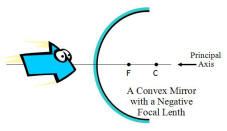800.608.6568 Buy On-Line# Convex Mirrors

## Seeing Images in a Convex Mirrors

We see images in a convex mirror much the same way we see images in a concave mirror.  Now we will look at the reflection of light in a convex mirror and how it produces real and virtual images depending on the objects location.  Just as with the page on concave mirrors, we will look at how convex mirrors reflect the rays of light and produce the images we see.The diagram at the right illustrates a convex mirror.  A convex mirror is described as a part of a sphere which had been sliced away.  If the outside of the sphere is silvered (shown here in blue) such that it can reflect light, then the mirror is understood to be convex.  The center of that initial sphere, before it was sliced, is known as the center of curvature (C) and the line which passes from the mirror's surface through the sphere's center is known as the principal axis.  The mirror has a focal point (F) which is located along the principal axis, halfway between the mirror's surface and the center of curvature (C). Note that the center of curvature and the focal point are located on the side of the mirror opposed to the object - behind the mirror.  Because the focal point is located behind the convex mirror, such a mirror is said to have a negative focal length value.